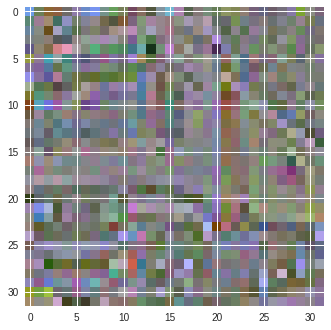# How to apply the ZCA whitening matrix correctly with LinearTransformation

I am trying to apply ZCA whitening matrix to my dataset. The code I use is as follows:

``````
import torchvision
import torch

import torchvision.transforms as transforms
from torchvision import transforms, datasets, models
import matplotlib.pyplot as plt
import numpy as np

def show(i):
i = i.reshape((32,32,3))

m,M = i.min(), i.max()
plt.imshow((i - m) / (M - m))
plt.show()

def computeZCAMAtrix():

#This function computes the ZCA matrix for a set of observables X where
#rows are the observations and columns are the variables (M x C x W x H matrix)
#C is number of color channels and W x H is width and height of each image

root = 'cifar10/'

temp= datasets.CIFAR10(root = root,
train = True,

#normalize the data to [0 1] range
temp.train_data=temp.train_data/255

#compute mean and std and normalize the data to -1 1 range with 1 std
mean=(temp.train_data.mean(axis=(0,1,2)))
std=(temp.train_data.std(axis=(0,1,2)))

#reshape data from M x C x W x H to M x N where N=C x W x H
X = temp.train_data
X = X.reshape(-1, 3072)

# compute the covariance
cov = np.cov(X, rowvar=False)   # cov is (N, N)

# singular value decomposition
U,S,V = np.linalg.svd(cov)     # U is (N, N), S is (N,1) V is (N,N)
# build the ZCA matrix which is (N,N)
epsilon = 1e-5
zca_matrix = np.dot(U, np.dot(np.diag(1.0/np.sqrt(S + epsilon)), U.T))

return (torch.from_numpy(zca_matrix).float(), mean, std)

#this transformation is used to transform the images to [0,1] range
#then normalize to 0 mean and 1 std, and then some
#random transformation to boost data variety at each epoch

batch_size=4

(Z,mean,std) = computeZCAMAtrix()
root = 'cifar10/'

transform_train = transforms.Compose(
[
transforms.ToTensor(),
transforms.LinearTransformation(Z),
])

transform_test = transforms.Compose(
[     transforms.ToTensor(),
transforms.LinearTransformation(Z),
])

#get the training and test sets
training_set = datasets.CIFAR10(root = root,
transform = transform_train,
train = True,

test_set = datasets.CIFAR10(root = root,
transform = transform_test,
train = False,

#trying to apply the transformation manually to see the result it produces
X = training_set.train_data
X = X.reshape(-1, 3072)
a=X
zca2=np.dot(a,Z)
print('printing truck')
show2(zca2)

batch_size=batch_size,
shuffle=False
)

batch_size=batch_size,
shuffle=False

)

classes = ('plane', 'car', 'bird', 'cat',
'deer', 'dog', 'frog', 'horse', 'ship', 'truck')
# get some random training images

for i, (images, labels) in enumerate(training_loader ,1):

a=images[0,:,:,:]
b=images[1,:,:,:]
c=images[2,:,:,:]
d=images[3,:,:,:]

#show images
#imshow(torchvision.utils.make_grid(images))

print('%5s' % classes[labels])
show(b.numpy())
break

``````

As you see I compute the ZCAmatrix and feed it as input (Z) to the dataloading function. However in computation of the ZCA matrix I assume that the data is of the form 50000 x H x W x C. However if I want to apply it as a transformation during dataloading with transforms.LinearTransformation(Z), it seems I need to first convert it to tensor using ToTensor which reorders data as 50000 x C x H x W. Then the application of the ZCA matrix to the data points reorder and flattened this way does not produce what I want it to produce. For instance if I manually apply the transformation to a truck picture of the form H x W x C I get the first picture below where as the LinearTransformation produces the second picture.

How can I work around this? it also seems I need to produce the ZCA matrix using 50000 x H x W x C as reordering data the other form and computing the ZCA matrix does not produce correct results as well.You can find out how to use ZCA in PyTorch here.

how to do this?
we apply ZCA on the whole dataset or we apply ZCA on everyt batch?
I just feel so confused

1 Like